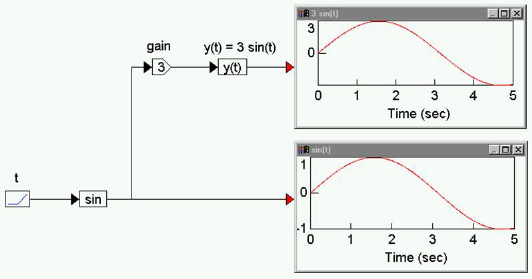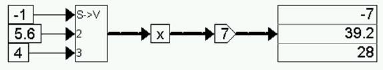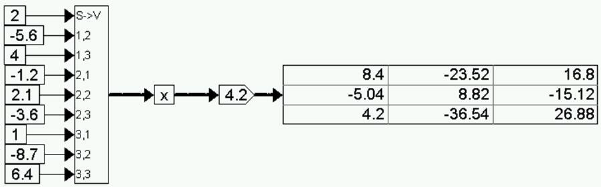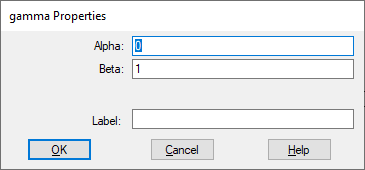## absBlock Category: Arithmetic

Inputs: Real, complex, or fixed-point scalars, or vectors or matrices.

Description: The abs block produces the absolute value of the input signal.Label: Indicates a user-defined block label that appears when View > Block Labels is activated.

#### Examples

1. Absolute value of a scalar

Consider the equation y = abs (sin (t)) realized as:The results in the two plot blocks show that the abs block computes the absolute value of the input signal.

2. Absolute value of a vector

Consider the equation:

w = abs (x)

where x = [-7 1 -2.2] realized as:When the simulation runs, the abs block computes an element-by-element absolute value of the vector x.

3. Absolute value of a matrix

Consider the equation:

Z = abs(Q)

where:This equation is realized as:Four const blocks provide the vector element values of Q through a scalarToVec block. When the simulation runs, the abs block computes the element-by-element absolute value of the incoming matrix.